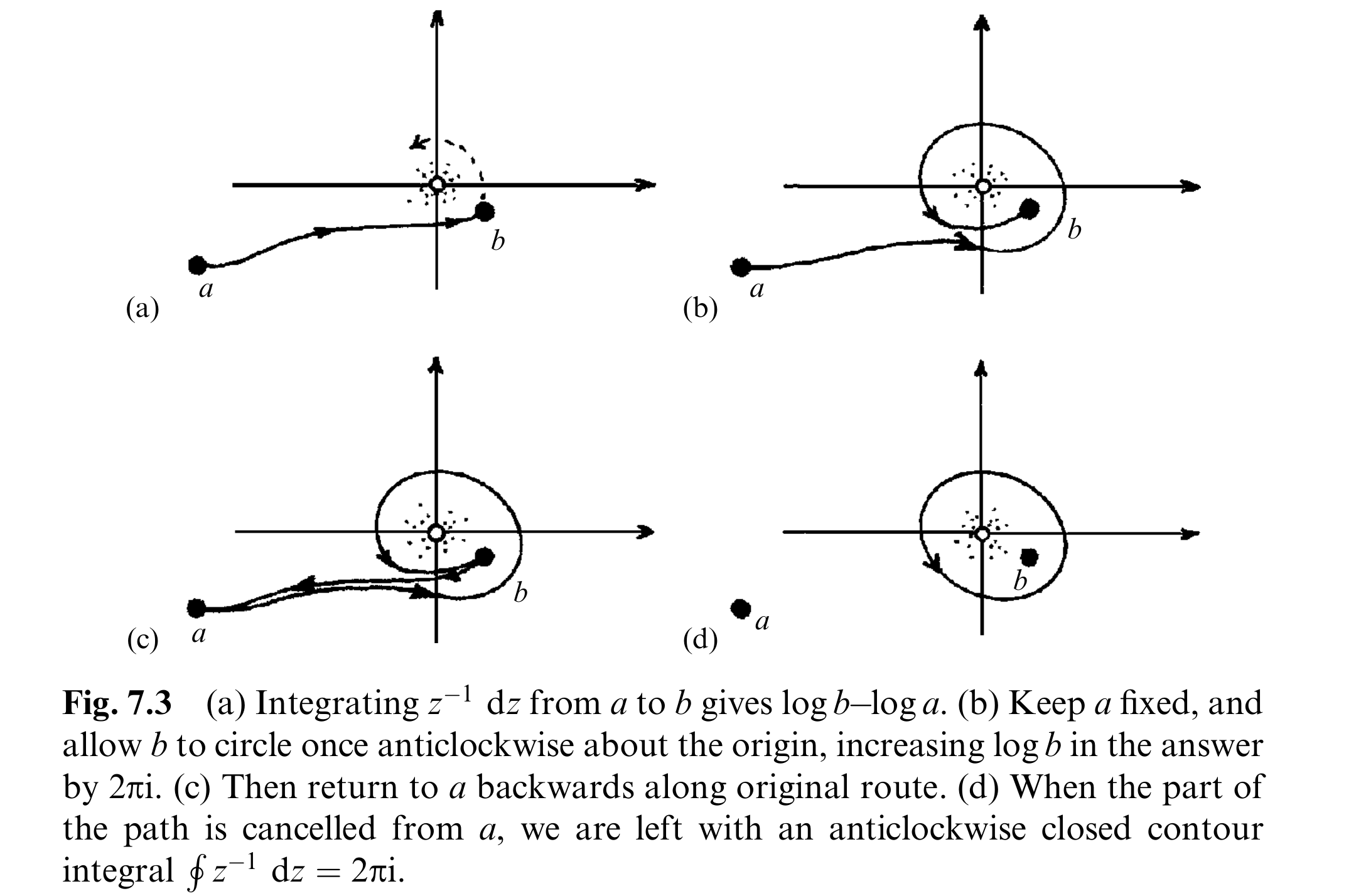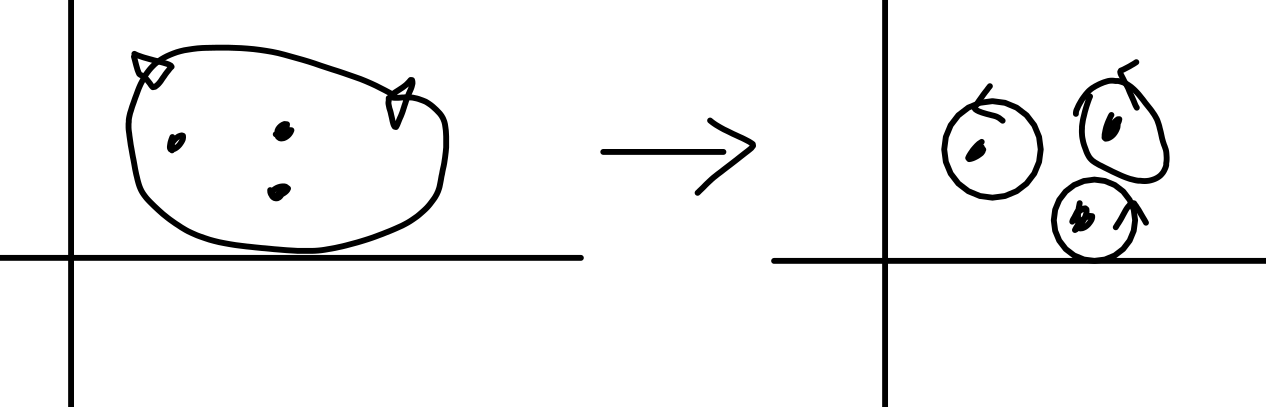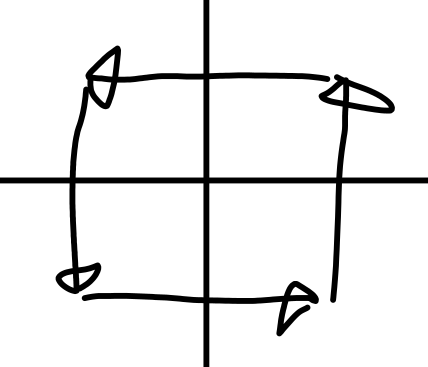### Cauchy Theorem

In general we have

$\frac{n!}{2\pi i} \oint \frac{f(z)}{(z-p)^{n+1}}dz = f^{(n)}(p)$

Which can be proven from the power series of $f(z)$ and the fact that $\oint 1/z = 2\pi i$ while $\oint 1/z^n = 0$. (True by loop arguments, see figure below)The interesting bit is that defining the taylor coefficients like this also works, meaning a smooth complex function has a power series! You prove this with cursed analysis.

### Residue theorem

A residue takes a pole $\alpha$ and writes

$f(z) = \frac{h(z)}{(z-\alpha)^n}$

Where $h(z)$ is regular, and this equality holds in some circle around $z = \alpha$. The residue is then defined as

$\frac{h^{(n-1)}(\alpha)}{(n-1)!}$

The residue theorem is that

$\oint_r f(z)dz = 2\pi i \;\times \; \left\{\text{sum of residues}\right\}$

Prove this by contracting around each pole to getWhich, after writing $f(z) = \frac{h^{(n-1)}(\alpha)}{(z-\alpha)^n}$ around each pole $\alpha$ and summing gives

$\oint_r f(z)dz = \oint \left\{\sum_{i=1}^{n} \frac{h_i(z)}{(z-\alpha_i)^{n_i}}\right\}dz = 2\pi i \left\{\sum_{i=1}^n \frac{h_i^{(n_i-1)}(\alpha_i)}{(n_i-1)!}\right\}$

### 7.6

We're going to show $\sum 1/n^2 = \pi^2/6$ by computing a contour integral around the $2N+1$ box, then letting $N \to \infty$.First use the residue theorem on

$\oint z^{-2}\cot{(\pi z)}dz$

There's a pole at every $z \in \mathbf{Z}$, first compute the residues. Consider $z = 0$ and $z \in \mathbf{Z}\setminus \{0\}$ seperately.

Let $\alpha \in \mathbf{Z} \setminus \{0\}$ be a pole. We can write

$f(z) = z^{-2}\cot(\pi z) = \frac{h(z)}{(z - \alpha)}$

For regular $h(z)$. In other words $h(z) = \frac{z-\alpha}{z^2}\cot(\pi z)$. Computing the residue is $h^{(0)}(\alpha)/0! = \lim_{z \to \alpha} h(z)$ which, using LHopital's rule gives

$\lim_{z \to \alpha} \frac{z-\alpha}{z^2} \frac{\cos(\pi z)}{\sin(\pi z)} = \lim_{z \to \alpha} \frac{1}{\pi z^2} = \frac{1}{\pi \alpha^2}$

(This is where we get the $1/n^2$ in the sum from!)

Now consider the pole at $z = 0$. It's multiplicity $3$ ($2$ from $z^{-2}$ and $1$ from $\cot(\pi z)$) meaning we can write

$h(z) = \frac{z^3 \cot(\pi z)}{z^2} = z\cot(\pi z)$

Computing the residue means computing $h^{(2)}(0)/2!$, taking derivatives gives

$\frac{h^{(2)}(z)}{2!} = \pi(\pi z\cot(\pi z) - 1)\csc^2(\pi z)$

As $z \to 0$ the limit goes to $-\pi/3$ (source: trust me bro.) meaning the integral (by the residue theorem) equals

$\oint z^{-2}\cot{(\pi z)}dz = 2\pi i\left(-\frac{\pi}{3} + 2\sum_{i=1}^{N} \frac{1}{\pi n^2} \right)$

If we can just show this integral goes to zero as $N \to \infty$ that'll imply

$\sum_{i=1}^N \frac{1}{n^2} \to \frac{\pi^2}{6}$

We show the integral goes to zero by bounding the integrand via a constant, since all constants have zero contour integral. It's possible to show

$|\cot(\pi z)| \le 2 \quad\text{(for large N and z on the 2N+1 box boundary)}$

Using Eulers formula and some algebra. First use the formula

$\cot(\pi z) = \frac{e^{i \pi z} + e^{-i\pi z}}{e^{i\pi z} - e^{-i\pi z}} = \frac{1 + e^{-2\pi i z}}{1 - e^{-2\pi i z}}$

Then set $z = (N+1/2) + iw$ for the vertical ones, we have (separating real and imaginary parts)

\begin{aligned} \cot(\pi (2N+1) + iw) &= \frac{1 + e^{-2\pi i(N+1/2)}e^{-2\pi i(iw)}}{1 - e^{-2\pi i(N+1/2)}e^{-2\pi i(iw)}} \\ &= \frac{1 - e^{-2\pi i(iw)}}{1 + e^{-2\pi i(iw)}} \\ &= \frac{1 - e^{2\pi w}}{1 + e^{2\pi w}} \end{aligned}

At this point you either realize this equals $\tanh(2\pi w)$ and so is bounded in norm by $1$, or you see that

$\left|\frac{1 - e^{x}}{1 + e^x}\right| = \left|\frac{2}{1 + e^x} - 1\right| < 1$

Now for the horizontal ones set $z = w + i(N+1/2)$ and simplify the exponents

\begin{aligned} \cot(\pi z) &= \frac{1 + e^{-2\pi iw}e^{2\pi (N+1/2)}}{1 - e^{-2\pi iw} e^{2\pi (N+1/2)}} \end{aligned}

The $e^{-2\pi i w}$ has norm $1$, and as $N \to \infty$ the $e^{2\pi (N+1/2)}$ terms dominate, meaning the ratio goes to $1$, meaning for all $\epsilon > 0$ there exists an $N$ large enough that

$\left| \frac{1 + e^{-2\pi iw}e^{2\pi (N+1/2)}}{1 - e^{-2\pi iw} e^{2\pi (N+1/2)}}\right| \le 1 + \epsilon$

Setting $\epsilon = 1$ and combining this with out previous result gives

$\left|\frac{\cot(\pi z)}{z^2}\right| \le \frac{2}{N^2}$

Which multiplied by the perimeter $4(2N+1)$ gives an upper bound for the path integral around the boundary (which is the contour integral).

$\left|\oint z^{-2}\cot(\pi z)dz\right| \le \left|\int_P \frac{2}{N^2}dz\right| \le \left(\frac{2}{N^2}\right)\left(8N+4\right) \le \frac{20}{N}$

This goes to zero as $N \to \infty$